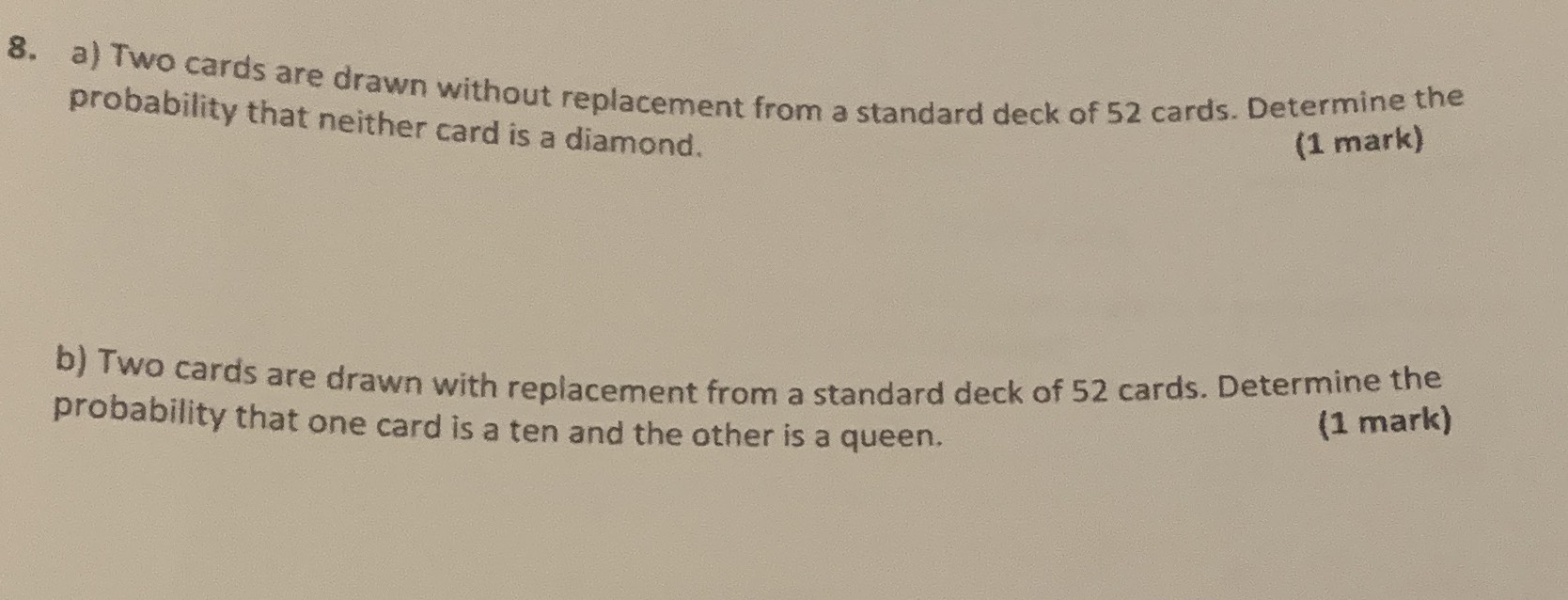### ¿Todavía tienes preguntas de matemáticas?

Pregunte a nuestros tutores expertos
Algebra
Pregunta8. a) Two cards are drawn without replacement from a standard deck of $$52$$ cards. Determine the probability that neither card is a diamond. (1 mark)

b) Two cards are drawn with replacement from a standard deck of $$52$$ cards. Determine the probability that one card is a ten and the other is a queen. (1 mark)

a) $$\frac{248}{663}$$
b)$$\frac{8}{663}$$